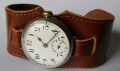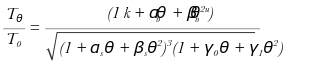Vintage Watchstraps

Straps for Vintage Fixed Wire Lug Trench Watches or Officer's Wristwatches

# Equations

## Equation 5

TeX Code rendered by Browser, style="font-size:200%;"

$$T=2\pi \sqrt\frac{12mk^2l}{t^3E}$$

SVG image: Baskerville italic, Unicode superscriptsSVG image from TeX code## Equation 7

TeX Code (with spaces around +) rendered by Browser.

$$\frac{T_\theta}{T_0} = \frac{(1\,+\,\alpha_b\theta\,+\,\beta_b\theta^2)}{\sqrt{(1\,+\,\alpha_s\theta\,+\,\beta_s\theta^2)^3(1\,+\,\gamma_0\theta\,+\,\gamma_1\theta^2)}}$$

SVG image: Baskerville italic, Unicode superscripts. The Chrome browser puts the Unicode superscripts at the correct height; Firefox and Microsoft Edge don't. Which is really weird, because Firefox and Edge put the superscripts in the correct position for equation 5 above!SVG image: Baskerville italic, Unicode superscripts - testing Kerning in Inkscape: some effect on alpha-theta spacing, no apparent effect on beta-theta spacing. The extra k and u are there because without them I wasn't convinced that any changes were taking place in the SVG file!PNG image: Baskerville italic, Unicode superscripts - testing Kerning in Inkscape: it works, but ugh! The image below is a PNG version of the SVG image above; no changes at all, just saved in the different format. The Greek letters appear the same as they do in Inkscape, whereas the browser renders them completely differently.SVG image from TeX codeTeX Code (with spaces around +) rendered by Browser

$$\frac{T_\theta}{T_0} = \frac{(1\,+\,\alpha_b\theta\,+\,\beta_b\theta^2)}{\sqrt{(1\,+\,\alpha_s\theta\,+\,\beta_s\theta^2)^3(1\,+\,\gamma_0\theta\,+\,\gamma_1\theta^2)}}$$

If you have any comments or questions, please don't hesitate to get in touch via my Contact Me page.